Impact Factor 3.560 | CiteScore 3.1
More on impact ›

# Frontiers in Physics## HYPOTHESIS AND THEORY article

Front. Phys., 08 February 2019 | https://doi.org/10.3389/fphy.2019.00005

# Precession Intuitively Explained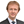Péter Hantz1* andZsolt I. Lázár2
• 1Department of Laboratory Medicine, Medical School, University of Pécs, Pécs, Hungary
• 2Faculty of Physics, Babes-Bolyai University, Cluj-Napoca, Romania

Formal explanations of the dynamics of free and heavy tops are considered to be known for centuries. However, intuitive understanding of their unexpected behavior is still a challenge. In this paper, we present elementary force based explanations of precession of heavy symmetric tops, derived in inertial and non-inertial reference frames. Simple calculations are enabled by a model equivalent to a spinning top, namely a square-formed tube carrying a streaming ideal fluid or a frictionless chain. Precession in a combined, gravitational and magnetic field is also discussed.

## 1. Introduction

Interpreting the wobble of a torque-free spinning plate, the rise of a tippe top, as well as the precession of a fast spinning wheel suspended at the extremity of its axle and exposed to gravitational or electromagnetic torque, inspired the brightest researchers during the past centuries. Although the mathematical treatment of these phenomena is known, their intuitive understanding is not trivial . Some mainstream textbooks provide simplified experimental configurations (e.g., dumbbells) to offer a more comprehensible explanation [7, 8]. However, none of these reveals the interplay of the forces responsible for these counterintuitive behaviors. Such a description would be of pivotal importance, since forces, in contrast to conservation laws, are closer to our everyday experience. Deeper understanding of torque-induced precession would be particularly important for teaching a series of its applications, like the gyroscope, electron spin resonance (ESR)  and nuclear magnetic resonance (NMR) [10, 11]. Moreover, the mathematical frameworks describing the motion of spinning tops and that for the equilibrium shape of idealized bent and twisted rods are analogous. This similarity is referred to as the Kirchhoff kinetic analogy (see Supplementary Section III).

Here we provide an intuitive and simple explanation of the torque-induced precession. After a short recapitulation of the underlaying concepts, we introduce and quantify the square wheel model. Our model relies on a square-shaped rigid tube with a heavy fluid or chain in its interior, which is allowed to circulate smoothly and without resistance in the closed loop determined by the rigid tube. This flow generates an angular momentum enabling the comparison of the novel configuration to the classical heavy tops which are symmetric, spinning objects. More importantly, the square-shaped tubing facilitates an intuitive and elementary quantification of the external couple of forces (in the inertial, laboratory frame) or the couple of inertial forces (in two types of rotating frames) which counteract the gravitational torque and prevent the top from tumbling down.

Spinning symmetric tops are prolonged or oblated bodies with a cylindrical or discrete rotational symmetry, whirling around their symmetry axis en, having one point fixed, or at least restricted to move on a surface . The symmetry axis coincides with the principal axle having the smallest or highest moment of inertia, while the two further principal moments of inertia associated with the perpendicular principal axes (e) have the same magnitude. One of the characteristic phenomena exhibited by spinning tops is precession. This term is used to refer to several phenomena having different physical origins. Conversely, in different fields of science and engineering, the very same event may be called precession, wobbling or nutation [7, 8, 13, 14].

Torque-free precession occurs when no external couple of forces is acting on the top; its symmetry axis will sweep around the mantle of a cone with opening angle ϑ, hereinafter nutation angle, while the total angular momentum L of the system, which determines the axis of the cone, is conserved  (see Supplementary Section II.B). The mainstream physics literature refers to the rotation around the precessing symmetry axis as spinning while to the rotation around the steady axis defined by the angular momentum as precession. Note that different points of a freely precessing body are subjected to acceleration which varies in space and time, therefore mechanical tensions will arise within precessing free tops. This phenomenon has a major importance in planetary physics  (see Supplementary Section II.D).

Torque-induced precession (Figure 1) takes place if a spinning top is exposed to a couple of forces (also referred to as moment or torque) [7, 2230]. Tops with an axis having a cylindrical or at least a third order discrete rotational symmetry, spinning around this axis and exposed to a torque follow a regular trajectory (see Supplementary Section II.E). Here the smooth change of the precession angle of the spinning (and symmetry) axis, that is, the precession, can be accompanied by nutation, a regular “nodding" of the nutation angle. It is important to mention that asymmetric tops will typically show chaotic behavior . Presence of dissipation can lead to exotic dynamics like the inversion of the tippe top [5, 32]. We only investigate the pure precession (steady nutation angle) of symmetric tops exposed to a torque (“heavy” tops), where dissipation is neglected.

FIGURE 1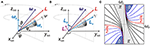Figure 1. (color online) Precession of a heavy symmetric top. (A) focuses on angular frequencies, while (B) on angular momenta. The index lab specifies the axis of the laboratory (inertial) reference frame. Quantities associated with precession are marked by p, those with spinning by s, and the ones related to the symmetry axis and the principal axes perpendicular to it, by n and ⊥, respectively. (C) Interdependence of the orientation ϑ, precession ωp, and spin ωs of a heavy top, described by Equation (1). The lines represent constant nutation angle (ϑ) curves. Intersections of the curves with a horizontal line show the allowed value(s) for the precession angular frequency ωp. Note that for ϑ∈{0, π} ωp and ωs cannot be distinguished .

### 1.1. Giroscopic Precession Essentials

Depending on the initial conditions and parameters, torque-induced (gyroscopic) precession can show dynamics with various complexity. Accordingly, its mathematical description demands tools of different levels of advancement [7, 22, 3335].

In the mainstream approach the classical spinning top is parameterized by the Euler angles, namely the nutation angle ϑ, the precession angle φ, and spin angle, ψ (Figure 1A). Without restricting generality, we can assume that the top is suspended in a pivotal point such that it can rotate freely, and the torque is of gravitational origin, and the corresponding force acts along the vertical axis. The nutation-free case, when ϑ is constant, can easily be treated by the Newton-Euler equation in the laboratory frame . This relationship states that the torque N acting on a top determines the rate of change of its total angular momentum L, namely dL/dt = N. The symmetry axis of the top will revolve around the vertical by a uniform precession angular frequency, ωp = /dt [37, 38], while the spinning angular frequency is ωs = /dt.

Deriving the equations of the general motion for the symmetric heavy top can be accomplished by several approaches. Besides the torque method relying on the Newton-Euler equations, Euler's equations or the toolkit of the analytical mechanics can also be applied [29, 30, 39]. Most derivations use a special decomposition of the angular momentum and angular frequency vectors. For the nutation-free case, these lead to a relationship involving ωp, the gravitational torque mgH, the principal moments of inertia In and I, as well as ϑ and ωs, namely

where m is the mass of the top, H is the distance of the top's center of mass from the pivotal point and the inertial moments are also calculated relative to this point  (see also Supplementary Figure 5).

The interplay of the parameters can lead to various behavior. The quadratic nature of (1) would generally yield two solutions in ωp. Figure 1C summarizes the main features of this equation . Note that this phase diagram can be “palpated” by a heavy top where the spinning and precession angular frequencies are independently controlled, while the nutation angle is free to alter .

For a prolated top, i.e., I > In, one can read from Figure 1C that for ϑ < π/2 (the symmetry axis “points upwards”) no dynamically stable solutions exist below a critical value of ωs (or ωp). If two solutions exist, they are referred to as “slow” and “fast” precession [23, 25]. For ϑ > π/2 the different signs of the two solutions represent precessions in the two opposite directions. The ϑ = π/2 case leads to a linear equation with a unique solution.

An approximate linear solution can be achieved for 0 < ϑ < π/2 range if assuming that the angular momentum of the precession, Lp (or equivalently, L) gives a negligible contribution to the total angular momentum L (see Figure 1B). More precisely, we neglect its contribution to the alteration of L, that is, dLdLs = ωsInden. This common approximation holds in the limit of large spin frequencies when ωsp ≫ 1 enabling to neglect the first term on the left of (1), and yielding a single “slow precession" solution ωp = mgH/Ls = mgH/In ω s.

In the general case nutation, that is, the periodic variation of the nutation angle, ϑ, will also occur. Although Newtonian methods could also account for nutation , this phenomenon can be less tediously treated by recurring to the toolkit of analytical mechanics [24, 30, 39, 42] finally leading to equations with elliptic functions .

### 1.2. Attempts for Intuitive Explanations So Far

Precessing heavy tops show several dynamical features which may seem paradoxical at a first glance (see Supplementary Section II.F). There have been several attempts for an intuitive explanation of the torque-induced precession. However, none of them provides a simple and easily quantifiable explanation of the “mysterious" torque which counteracts the external one. The main point of our paper is to present an almost trivial and intuitive model of the pure, torque-induced precession based on forces instead of conservation laws.

An early effort made to interpret the torque-induced precession is presented in the seminal book of Klein and Sommerfeld . They introduced the concept of “deviation resistance" (also referred as deviation torque), that is the torque counteracting the external (gravitational) one, and determined its connection to the centrifugal and Coriolis forces . Feynman proposed an explanation by observing that the paths obtained by projecting the trajectories of the point of a spinning and precessing top onto the horizontal plane swept by the symmetry axis. These paths are curved, what requires the presence of pairs of radial forces , but the idea has not been quantified (for an analytic and numeric approach, see the Supplementary Section I.A).

For a simple case, namely four identical masses on a crossbar, Barker calculated the change of the orientation of their velocities resulting from combined spinning and precession, and determined the overall torque required by the corresponding accelerations [48, 49]. Snider computed, using Coriolis forces, the torque distribution and the overall torque required to make a spinning ring to precess . Usubamatov derived the distribution of centrifugal forces, and their overall torque counteracting gravity for a spinning and precessing disk .

Widely used mainstream textbooks also struggle to offer some intuitive approaches. For example, Morin  and Kleppner and Kolenkow  analyze the effect of impulses applied to a dumbbell or to a spinning top in free fall. However, all of the attempts listed above either rely on pretty laborious calculations, or do not catch some important aspects of the precession. As such, the need for an intuitive and easy-to-quantify model, accounting for the most relevant aspect of precession, has not yet been fulfilled.

## 2. The Square Wheel Model

### 2.1. Model Outline

The dynamic equilibrium of a spinning and precessing top will be discussed by determining the forces and torques acting on it, rather than considering the Newton-Euler equation. Performing quantitative investigations would be simpler if very special top shapes could be considered. The rigidity of the classical top limits choosing the right geometry. Let us, however, substitute the spinning wheel (Figure 2A) by the flow of an ideal and incompressible fluid with streamlines strictly parallel to a closed and rigid tube, or by an ideally flexible, frictionless chain running along a fixed and closed trajectory (Figures 2B,C). In this way, we can generate the same angular momenta as with a wheel, while a flow or chain loop can be reshaped such that it greatly simplifies calculations (see Supplementary Section I.B). The dynamics of the classical top and our proposed square wheel arrangement are uniquely determined by the torque acting on them, and their angular momentum vector. Therefore the systems can be regarded as being dynamically equivalent if the torques and angular momenta for the two systems are the same. The angular momentum has two components associated with the two types of motion: circulation about a symmetry axis and rotation about a fixed vertical axis. Note that the shape of the proposed system, which determines the trajectory of the ideal chain or fluid, is also fixed. However, for a noncircular hoop, unlike for the rotationally symmetric rigid bodies, the distances between the symmetry axis and the mass elements moving on a closed trajectory are not constant. As such the above hybrid model combines a rigid support and an ideally flexible component generating the angular momentum, the fluid or chain.

FIGURE 2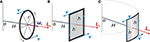Figure 2. (color online) Simple models of a heavy top. v represent the flow velocities relative to the center of mass. (A) Spinning wheel with an angular momentum ${L}_{s}=m{r}^{2}{\omega }_{s}$ where v = s (B) Square wheel with an angular momentum Ls = mvh generated by a heavy, ideal fluid flowing, or an ideally flexible, frictionless chain running in a square-shaped hoop. This model allows the straightforward analytic treatment of the problem in inertial and precessing reference frames. (C) Bent square wheel model of a heavy top providing circular trajectories for the chain or fluid particles in the upper and lower segments of the hoop. This approach allows a simple intuitive understanding of the precession in inertial and body (connected to the fluid/chain) reference frames.

This model has been introduced in 1945 by Rood  but was forgotten by the Physics community, and has been re-discovered later . Up to this time, inertial properties of liquids circulated in tubings of various shapes were explored only for technological purposes, namely, measuring liquid flow speed [54, 55].

Let us consider a wheel with radius r, whose mass m is entirely concentrated in a thin hoop (Figure 2A). When spinning with angular frequency ωs (without rotating around the vertical shaft), the wheel has a spinning angular momentum of ${\text{L}}_{s}={r}^{2}m{\omega }_{s}{\text{e}}_{n}$, where en is the unit vector along the axis of the wheel (Figure 1A). Obviously, the angular momentum would not change if the wheel did not spin, but the heavy hoop was replaced by a massless tube containing an ideal fluid or frictionless chain flowing with v = s and having mass m (see Supplementary Section I.A). Moreover, the circular tube could be reshaped into a square (Figure 2B).

If the hoop -with the circulating fluid or chain- rotates around the vertical (e.g., it precesses), the total angular momentum will acquire a component Lp along the z-axis, therefore its total angular momentum reads L = Ls + Lp.

This shape enables the simple featuring of the forces acting on it during precession, and the subsequent calculations will also become technically less demanding. It can easily be shown that a square wheel having side length 2h, containing an ideal fluid or chain with mass m and flow speed v, has an angular momentum Ls = mvh with respect to its center .

Some aspects of the model can further be simplified if we consider a square wheel bent in a way that its upper and lower segments form arcs of radius H (Figure 2C). For example, in the case of a precessing (rotating around the vertical axis) flat square wheel, the chain/fluid elements will follow complicated cycloidal orbits. Such orbits can even lead to outward-pointing centripetal forces (see Supplementary Figure 1). For a precessing bent square wheel with the curvature radius equaling the axis length H, only circular orbits are allowed for the circulating mass elements in the horizontal hoop segments, and therefore the centripetal force will always point inward. The angular momenta can be calculated for various shapes using Stokes' theorem (see Supplementary Section I.C).

Interpretation of the forces present in the system depends on the choice of the reference frame. A comparative analysis of the forces and torques in the laboratory (inertial), as well as in two kinds of rotating (non-inertial) reference frames will be considered, where different real and inertial forces play a role. In this paper, we will restrict ourselves to the nutation-free and ϑ = π/2 case. Thus, the nutation angle is constant, the spin angle is irrelevant for the square wheel model (the movement of the chain or fluid elements is characterized by their speed), and only the precession angle has a role of a dynamic variable. In order to present easy analytic calculations or intuitive explanations in different reference frames, we will use the flat and the bent square wheel models as well. While analytical calculations are facilitated by the flat geometry, within a qualitative, intuition based approach the role and action of forces are easier to grasp in the bent square wheel model (see section 2.5). We assume that the rigid support of the fluid or chain including the axis touching the suspension point O are massless.

Note that the forces arising at the corners of the square wheel, making the circulating chain or fluid elements change direction, are internal forces with reflection symmetries. Therefore they can be disregarded while describing the global dynamics of the system.

### 2.2. Preliminaries

First, we recapitulate the forces acting on a material point which is fixed or is moving on a horizontal and rotating platform. We also consider the forces acting on the platform itself. If there is no vertical acceleration, the gravitational force G acting on the material point, and the supporting force acting from the support back on the material point, cancel (see Supplementary Figures 10 and 11).

In the laboratory (inertial) reference frame only real forces are present. The material point is accelerated (kept on a circular orbit) by the centripetal force FCP. The action-reaction pair of the centripetal force will be referred to as the reactive central force, RC. This force represents the action of the accelerating body on the platform.

In the non-inertial, rotating reference frame attached to the platform, the forces acting on the body cancel. The centrifugal force, FCF, is a fictitious force acting on the body, which does not have an action-reaction pair. This is canceled by the real constraint force, TCF also referred to as the central force, which pulls the body toward the center. The action-reaction pair of TCF, acting on the platform, is the reactive central force RC.

If the material point is not fixed to the rotating platform, but forced to perform a rectilinear uniform motion with respect to the platform, the dynamics can be conveniently described from the non-inertial, rotating reference frame. In this frame, two inertial forces act on the body, namely the centrifugal, FCF, and Coriolis force, FCOR. These inertial forces are balanced out by real constraint forces referred to as TCF and TCOR. The action-reaction counterpart of these real forces, acting on the platform, are the reactive central force, RC, and the reactive Coriolis force, RCOR. The sum of these two forces can be referred as the reactive force R.

In the non-inertial, comoving reference frame attached to a non-axial point of the spinning top, or to a circulating mass element of the square wheel, the fictitious centrifugal forces FCF, their “canceling" real constraint forces TCF, as well as the action-reaction pairs RC are present. Note that the magnitude of these forces are not the same as for the rotating (precessing) reference frame.

Table 1 offers an overview of the reference frame dependent interpretation of the forces acting on, and the angular momentum of the square wheel system. In this table, the forces acting on the elements of the fluid or chain, as well as those acting on the support, are separately marked. Note that the net force (including the forces present in the pivotal (suspension) point O) and net torque acting on the support has to vanish, since the support itself is considered to be massless.

TABLE 1

### 2.3. Inertial Reference Frame, Intuitive Treatment

Conceptually, the inertial frame allows the simplest, intuitive explanation of the gyroscopic precession. A straightforward treatment can be conducted by computing the contribution of torques acting on the support. Since the support is assumed to be massless the net torque acting on it has to vanish.

Let us consider a bent square wheel, with the radius of curvature, H, equaling the length of the axis attached to it (see Figure 2). We study the configuration with the angular momentum of the top pointing outwards. As previously mentioned, in this paper we only treat the simple case when the symmetry axis of the top makes a right angle with the vertical (ϑ = π/2). Therefore the chain/fluid in the square wheel circulates such that in the upper segment its absolute velocity (measured in the laboratory frame) is reduced while in the lower is increased due to the precession marked by ωp.

The centripetal forces keeping the chain or fluid on the curved trajectory have larger magnitude in the lower, and smaller magnitude in the upper fluid or chain segment. Accordingly the reactive central force acting outwards on the lower support segment will also have a higher magnitude than the one acting, also outwards, on the upper segment (see Figure 3). Therefore, two external torques will act on the support: one, NG, originating from the weight of the chain or fluid, while the other one, NRC, is raised by two reactive central forces, having different magnitudes and acting on the upper and lower square wheel segments.

FIGURE 3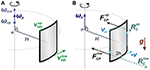Figure 3. (color online) The bent square wheel model, viewed from the laboratory (inertial) reference frame (see section 2.3). (A) The axis of length H precesses in the horizontal plane with angular frequency ωp. The velocities of the chain/fluid mass elements, measured from the laboratory frame (green arrows), have lower magnitude in the upper segment (where the speed of the chain/fluid elements subtracts from that of the surrounding support), and higher magnitude in the lower segment (where these speeds add up). (B) Constraint forces act on the chain/fluid elements (black continuous lines) while reactive forces act on the support (green dotted lines). Note the lower magnitude of the forces acting in the upper segment. Blue arrows represent the chain/fluid velocities measured from the precessing frame, which are identical around the support. Notation of forces (see section 2.2): FCP - centripetal, RC - reactive central. The pair of forces, RC, act on the support and generate the “lifting" torque counterbalancing the gravitational one.

The pair of reactive central forces will exert a torque on O which can counterbalance the torque of the weight. It can easily be shown that the side segments of the square wheel do not contribute to the “lifting" torque. This is the essence of the gyroscopic effect phrased in the inertial reference frame.

Note that the square wheel model also provides an intuitive understanding on the sequence of events happening when a spinning top hanged on its axis extremity is released. During the fall a pair of forces will arise in the vertical segments which will lead to a transient angular acceleration around the vertical and precession is initiated.

### 2.4. Inertial Reference Frame, Analytic Treatment

Simple analytic treatment of the problem from the inertial reference frame is allowed by the flat square wheel model (Figure 4C). We consider only the case when the nutation angle is right angle, that is, ϑ = π/2. Equilibrium conditions are computed considering the forces and torque acting on the support. The result of the calculation is the precession angular frequency.

FIGURE 4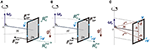Figure 4. (color online) Flat square wheel model of a heavy top in gravitational field with acceleration g, viewed from the laboratory (inertial) reference frame (see section 2.3). Absolute values of the chain/fluid velocities in the horizontal segments, with respect to the square wheel, are marked with blue. (A,B) Centripetal forces, FCP, acting on the fluid or chain in the middle of the horizontal segments marked by black continuous lines, reactive central forces, RC, acting on the corresponding support segments are marked by dotted green lines. Note that depending on the ratio of ωp and v, these can point in the same or in opposite directions (see also Supplementary Figure 1). (C) Sketch for the calculations of the torques raising in the symmetric points marked by rup and rlow (see Equations 2, 3).

For speed, acceleration and distance measured in the inertial (laboratory) frame we use the “lab” index. Note that plain v denotes the speed of the fluid/chain measured from the precessing reference frame.

The speed, ${\text{v}}_{lab}^{up/low}$, and centripetal acceleration, ${\text{a}}_{lab}^{up/low}$, of a chain/fluid mass element, dm, in the upper and lower segments at positions rup/low = H+δ±h, viewed from the laboratory frame reads ${\text{v}}_{lab}^{up/low}={\omega }_{p}×{\text{r}}^{up/low}±\text{v}$, while the centripetal accelerations are

Henceforth, when the ± (or ∓) signs appear in formulae pertaining to both the upper and lower wheel segments these are to be associated in order, i.e., + to upper, − to lower (or vice versa). Here we made use of the fact that the rate of change of the flow velocity v “bound" to the precessing wheel is:

pointing outwards, along the axis of the square wheel.

The force keeping a mass element on its trajectory is , while the force acting from the mass element on the support reads $d{\text{R}}_{C}^{up/low}=-d{\text{F}}_{CP}^{up/low}$ (see Figures 4A,B). The torque of the reactive forces exerted by chain/fluid mass elements with mass dm located in symmetric positions on the upper and lower support segments reads $d{\text{N}}_{{R}_{C}}^{up/low}=-dm·{\text{r}}^{up/low}×{\text{a}}_{lab}^{up/low}$. Since the symmetry of the square leads to the cancellation of the contributions through δ we have $d{\text{N}}_{{R}_{C}}^{up/low}=-dm·\left(±\text{h}\right)×\left[2{\omega }_{p}×\left(±\text{v}\right)\right]=-2dm·\text{h}×\left({\omega }_{p}×\text{v}\right)$, which is independent of the position along the horizontal segments. Finally, the overall torque acting on the support, obtained by integrating along the two horizontal segments, reads NRC = −m·h × (ωp × v). This torque points into a direction opposite to that of the gravitational torque NG = mH × g. The condition for the dynamic equilibrium of the support is NG + NRC = 0, that is, mgH = mhωpv, leading to

Here we mention that an alternative interpretation of the forces in the inertial frame, relying on the d'Alembert principle, may also be instructive. Note also that for the “tilted” configuration when ϑ≠π/2, the calculations become tedious, but some conclusions are summarized in Supplementary Section I.D.

### 2.5. “Comoving” (or “Fluid/Chain”) Reference Frame

In this approach, we compute the forces and torque acting on the upper and lower square wheel segments from the reference frames moving together with the circulating chain or fluid (for the case when the nutation angle is right, i.e., ϑ = π/2). The equilibrium condition demands vanishing net torque acting on the fluid or chain, that is NG + Ndev=0, where Ndev is the torque raised by the pair of centrifugal forces in the upper and lower square wheel segments, respectively. The precession angular frequency can immediately be obtained as before. The flat square wheel model enables an analytic solution leading to identical results to the one presented in section 2.4. Every circulating mass (fluid/chain) element will have an associated reference frame wherein the mass element is at rest. These individual comoving reference frames have different distances from, and orientations with respect to the precession axis. Centrifugal forces, as well as the corresponding reactive central forces have to be computed for all of these reference frames individually. This complicates the calculation of the net deviation torque.

In this case, though we have to settle with an approximation. It is more intuitive to apply for the bent square wheel model [21, 57] (Figure 2C). This circular setup facilitates the calculation of the individual centrifugal forces. However, for estimating the net torque we perform the approximation hH. In this case, when summing up the elementary centrifugal forces arising in the upper and lower segments, we consider them as being parallel to each other and the axis of the bent square wheel (Figure 5).

FIGURE 5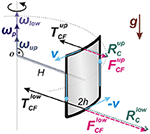Figure 5. (color online) Relevant forces in the bent square wheel model viewed from the comoving reference frames, that of the chain/fluid elements moving in the upper and lower horizontal square wheel segments (see section 2.5). Notation of forces (see section 2.2 for the nomenclature): FCF, centrifugal force; TCF, central force, both acting on the fluid or chain; RC, reactive central force acting on the support.

The angular velocities of the individual reference frames in the bent upper and lower segments are ωup/low = ωpv/H. If hH, the relevant component of the reactive central (and centrifugal) forces become ${F}_{CF}^{up/low}\approx \left(m/4\right){\left(v\mp {\omega }_{p}H\right)}^{2}/H$. The vertical fluid or chain segments will not contribute to the deviation torque. After adding the contribution from both horizontal segments, the net deviation torque will result in Ndevmhvωp. Since the equilibrium condition reads Ndev + NG = 0, this leads to the dynamic equilibrium condition expressed also by Equation (4).

### 2.6. Precessing Reference Frame

It is especially instructive to determine the torque in a reference frame precessing together with the center of mass of a flat square wheel (see Figure 6). In this frame, the velocities of the chain/fluid elements in the wheel segments will trace straight trajectories with a uniform speed. As a consequence, besides the centrifugal forces, Coriolis forces will also arise. The equilibrium sets when the net torque acting on the fluid or chain disappears, that is, Ndev + NG = 0. As we will see, only Coriolis forces in the upper and lower segments will contribute to the deviation torque.

FIGURE 6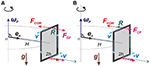Figure 6. (color online) Relevant forces in the flat square wheel model in the precessing reference frame (bound to the axis of the square wheel). Red broken lines denote the inertial forces acting on the fluid/chain, while green dotted ones the reactive forces acting on the support. (A) Lower flow speed, when the centrifugal force dominates over the Coriolis force, yielding an outward pointing net inertial force. (B) Higher flow speed, when the Coriolis force dominates over the centrifugal force, and an inward pointing net inertial force will result. Notation of forces (see section 2.2 for the nomenclature): FCF - centrifugal, FCOR - Coriolis, R - reactive (see section 2.6).

In the case of a flat square wheel having the nutation angle ϑ = π/2 the lateral and horizontal wheel segments have a symmetric arrangement. As centrifugal forces do not depend on speed their torques cancel. Therefore, only the Coriolis forces will contribute to the deviation torque. Their magnitude on the upper and lower segments is the same, but they point in opposite directions. Note that the net reactive force raising on these segments can point radially inwards or outwards as well, depending on the relative magnitude of the Coriolis and centrifugal forces.

For the vertical segments, the Coriolis forces are zero as the chain/fluid velocities are parallel to the rotation axis, ωp. In the upper and lower segments we have vωp and

where v stands for the flow velocity in the lower segment and en is the unit vector pointing from the pivotal point toward the center of the wheel. The deviation torque exerted on the suspension point O will therefore be

where ek = en × ez is horizontal and orthogonal to the symmetry axis. We obtain, without any approximation, the equilibrium condition expressed by Equation (4).

The precessing reference frame allows the analysis, with some approximation, of the “oblique” (ϑ≠π/2) case as well. Since the calculations are tedious, we just remark two important conclusions. First, the point of application of the resultant of the centrifugal forces will not be anymore the center of the square wheel. Second, surprisingly, the chain/fluid moving in the oblique square wheel segments will not contribute to the deviation torque counteracting the gravitational one. This finding allows the construction of a “top” consisting only of horizontal square wheel segments with a gas or a fluid streaming therein. Supplementary Figure 3 shows the schematic structure and functioning of a device that demonstrates the lifting torque of the forces arising in the horizontal segments.

### 2.7. Including Electromagnetic Interactions

The precession of bodies having, besides an angular momentum, an additional magnetic moment, bear special importance: the interaction of the magnetic moment with an external magnetic field will also contribute to the torque. Therefore, the precession will be altered compared to the purely gravitational case.

In the following, we examine a generalized square wheel model involved in both gravitational and electromagnetic interactions. Our model consists of a square wheel, where the chain/fluid flow having a velocity v carries an additional electric current I. This structure is placed in uniform magnetic B and gravitational g fields (see Figure 7).

FIGURE 7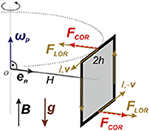Figure 7. (color online) Square wheel model where the chain/fluid flow having velocity v carries an additional electric current I. The system is placed in a combined magnetic B and gravitational g field, and investigated from the reference frame precessing with ωp. Besided the Coriolis forces FCOR, Lorentz forces FLOR also contribute to the canceling of the gravitational torque (see section 2.7).

We assume that switching to a reference frame rotating (precessing) with ωp the Lorentz force will not change (relativistic effects are neglected) . The calculations rely on the formal similarity between the expression of the Coriolis and the Lorentz force [59, 60].

The Coriolis force acting on a chain/fluid element reads dFCOR = −2·dm·ωp × v, while current elements in an external magnetic field are subject to a Lorentz force dFLOR = I·dl × B, where dl represents the length of the current element. Therefore, the sum of the Lorentz forces acting on the upper and lower square wheel segments will be ${\text{F}}_{LOR}^{up/low}=\mp 2hIB{\text{e}}_{n}$ (see Figure 7). Their torque exerted on the suspension point O can be expressed as NLOR = 2(2hIB)he = μBe, where μ = 4h2I = AI is the magnetic moment of the circuit, while A represents its area.

If we disregard nutation, the dynamic equilibrium establishes when the magnetic, deviation and gravitational torque cancel each other, that is $4{h}^{2}IB+m{\omega }_{p}vh-mgH=0$, which finally leads to

$ωp=mgH-4h2IBmvh=NG-μBLs=NG-NLORLs$

where NG is the gravitational torque. Note that in the case where μB = mgH, the magnetic and the gravitational torque may cancel each other even in the absence of angular momentum and precession.

## 3. Conclusion

In this paper we quantified the square wheel model of spinning tops. Although there were several attempts to find an intuitive, force-based explanation of the phenomenon of precession these approaches are not simultaneously simple, quantitative and intuitive [7, 8, 47, 48, 51, 52]. Circular tops do not allow easy calculations, dumbbells are oversimplified, while the first, forgotten paper of the square wheel model did not provide any quantification.

The proposed model consists of a heavy chain or fluid which frictionlessly circulates in a closed loop determined by a square-shaped tube, and therefore an angular momentum will arise. This model allows the explanation and quantitative treatment of gyroscopic precession by simply derived forces which maintain the top “against gravity.” These forces were interpreted in the laboratory (inertial), as well as in two types or rotating (non-inertial) reference frames. Our approach is more intuitive than the classical explanation relying on the Newton-Euler equation on the alteration of the angular momentum. We discussed an extended model as well, where the flow carries an electric current and an external magnetic field is also present besides the gravitational one. These thoughts contribute to the better and more intuitive understanding of the difficult, but intriguing problem of the precession of heavy tops, which is the ground for understanding various phenomena ranging from mechanical gyroscopes to magnetic resonance. Note that variants of the square wheel model can be applied to intuitively explain the dynamics of free tops as well (see Supplementary Sections II.I and J).

## Author Contributions

PH came to the idea of the square wheel, made the initial version of the calculations, prepared the figures, the referred patents, the numeric simulations, and equally contributed to the writing of the paper. ZL refined the calculations, performed the calculations in the Supplementary Material, and equally contributed to the writing of the paper.

## Funding

ZL is supported by the COFUND-FLAGERA II-CORTICITY grant.

## Conflict of Interest Statement

The authors declare that the research was conducted in the absence of any commercial or financial relationships that could be construed as a potential conflict of interest.

## Acknowledgments

We are indebted to A. Jakovác, Sz. Horvát, R. Németh, Gy. Lakos, Gy. Dávid, G. Györgyi, L. Orosz, P. Gnädig, and G. Domokos for useful discussions, while to Cs. András, J. Tellmann, S. Krueger-Lebus, T. Lessinnes, S. Bächtold, S. Habermacher, J. Spring, I. Jalsovszky and A. Málnási-Csizmadia for their help during the work. A. Jakovác and G. Györgyi provided substantial ideas for preparing Figure 1C and the explanation in the comoving reference frame. This work has been supported by the Domus Hungarica Foundation. The present scientific contribution is dedicated to the 650th Anniversary of the foundation of the University of Pécs, Hungary.

## Supplementary Material

The Supplementary Material for this article can be found online at: https://www.frontiersin.org/articles/10.3389/fphy.2019.00005/full#supplementary-material

## References

1. Simonyi K. The Cultural History of Physics. Boca Raton, FL: CRC Press (2012).

2. Arnold IV. Mathematical Methods of Classical Mechanics. New York, NY: Springer (1978).

3. Borelli A. Angular momentum between physics and mathematics. In: Schlote K-H, Schneider M, editors. Mathematics Meets Physics. Frankfurt: Verlag Harri Deutsch (2011). pp. 395–440.

4. Tuleja S, Gazovic B, Tomori A. Feynman's wobbling plate. Am J Phys. (2007) 75:240–44. doi: 10.1119/1.2402156

5. Cohen RJ. The tippe top revisited. Am J Phys. (1977) 45:12–7.

6. Zhu LY. Fun Rigid Body Dynamics. Beijing: Higher Education Press (2008).

7. Morin D. Introduction to Classical Mechanics, with Problems and Solutions. Cambridge: Cambride University Press (2007).

8. Kleppner D, Kolenkow R. An Introduction to Mechanics. Cambridge: Cambridge University Press (2014).

10. Hanson L. Is Quantum mechanics necessary for understanding magnetic resonance? Concepts Magn Reson A. (2008) 32:329–40. doi: 10.1002/cmr.a.20123

11. Magnetic Moments Interacting With Magnetic Fields. TeachSpin Inc. Avilable online at: www.teachspin.com

12. Brecher K. Top-ology: a torque about tops. In: Bridges Seoul Converence Proceedings (2014). pp. 51–8.

13. Tewari A. Atmospheric and Space Flight Dynamics. Boston, MA: Springer-Birkhauser (2007).

14. Dehant V, Mathews PM. Precession, Nutation and Wobble of the Earth. Cambridge: Cambridge University Press (2015).

15. Wolfram Demonstration project: Free precession of a Rotating Rigid Body. Available online at: demonstrations.wolfram.com/FreePrecessionOfARotatingRigidBody/

16. Butikov E. Inertial rotation of a rigid body. Eur J Phys. (2006) 27:913–22. doi: 10.1088/0143-0807/27/4/022

17. Elliott C. The Spinning Top. Durham (2009). Available online at: http://www.maths.dur.ac.uk/Ug/projects/highlights/CM3/Elliott_Spinning_Top_report.pdf

18. Tong D. Lectures on Classical Dynamics 3.: The Motion of Rigid Bodies. Available online at: www.damtp.cam.ac.uk/user/tong/dynamics.html

19. Tatum JB. Classical Mechanics. Available online at: astrowww.phys.uvic.ca/~tatum/classmechs

20. Lazarian A, Efroimsky M. Inelastic dissipation in a freely rotating body. Application to cosmic-dust alignment. Month Noti R Astr Soc. (1999) 303:673–684.

21. Jakovác A. Private Communication. Budapest (2013).

22. Berry MV, Shukla P. Slow manifold and Hannay angle in the spinning top. Eur J Phys. (2011) 32:115–27. doi: 10.1088/0143-0807/32/1/011

23. Wittenburg J. Dynamics of Multibody Systems. Berlin: Springer (2008).

24. Dreizler R, Lüdde C. Theoretical Mechanics. Berlin: Springer (2011).

25. Ginsberg J. Engineering Dynamics. Cambridge: Cambridge University Press (2008).

26. Georgi H, Yin X. Mechanics and Special Relativity. Harward Physics 16. Available online at: http://isites.harvard.edu/fs/docs/icb.topic1145127.files/Scanned%20topic%2011.pdf

27. Pritchard DE, et al. Three Dimensional Rotations and Gyroscopes. MIT Physics 8.01. Available online at: http://web.mit.edu/8.01t/www/materials/modules/chapter22.pdf

28. Provatidis C. Revisiting the spinning top. Int J Mat Mech Eng. (2012) 1:71–8. Available online at: http://www.seipub.org/ijmme/paperInfo.aspx?ID=2316

29. Landau L, Lifshitz EM. Mechanics (Vol. 1., Course of Theoretical Physics). Oxford: Pergamon Press (1969).

30. Kibble T, Berkshire F. Classical Mechanics. London: Imperial College Press (2004).

31. Barrientos M, Perez A, Ranada AF. Weak chaos in the asymmetric heavy top Eur J Phys. (1995) 16:106–12.

32. Moffatt HK. Euler's disk and its finite-time singularity. Nature (2000) 404:833–4. doi: 10.1038/35009017

33. Wolfram Demonstrations Project. Stationary Precession of a Spinning Top. Available online at: demonstrations.wolfram.com/StationaryPrecessionOfASpinningTopRevised/

34. Wilson HB, Turcoette LH, Halpern D. Advanced Mathematics and Mechanics Applications Using MATLAB. Boca Raton, FL: Chapman and Hall (2003).

35. Gander W, Hrebicek J. Solving Problems in Scientific Computing using Maple and MATLAB. Berlin: Springer (2004).

36. Precession on a Swivel-Chair. Available online at: www.youtube.com/watch?v=e9khBSG0zDk

37. MIT Physics Demo - Bycicle Wheel Gyroscope. Available online at: www.youtube.com/watch?v=8H98BgRzpOM

38. Gyroscope. Available online at: www.youtube.com/watch?v=-rXaFVYVMg0

39. Goldstein H, Poole Ch, Safko J. Classical Mechanics. San Francisco, CA: Addison-Wesley (2001).

40. Chouaieb N. Kirchhoff's Problem of Helical Solutions of Uniform Rods and Their Stability Properties. Thèse 2717, EPFL, Lausanne (2003).

42. Heard WB. Rigid Body Mechanics: Mathematics, Physics and Applications. Weinheim: Wiley-VCH (2005).

43. Lawden DF. Elliptic Functions and Applications. New York, NY: Springer (1989).

44. Klein F, Sommerfeld A. The Theory of the Top, Vol. I. Boston, MA: Birkhäuser (2008).

45. Scarborough JB. The Gyroscope Theory And Applications. New York, NY: Interscience Publisher (1958).

46. DeCaria AJ. The Coriolis and Centrifugal Torques in Gyroscopic Precession. Available online at: http://snowball.millersville.edu/~adecaria/DERIVATIONS/Coriolis_Torque.pdf

47. Feynman R, Leighton R, Sands M. The Feynman Lectures on Physics. Reading, MA: Addison-Wesley (1963).

48. Barker E. Elementary analysis of the gyroscope. Am J Phys. (1960) 28:808–10.

49. Solving the Mystery of Gyroscopes. Available online at: www.youtube.com/watch?v=TUgwaKebHTs

50. Snider JL. Gyroscopic precession. Am J Phys. (1965) 33:847.

51. Usubamatov R, Harun A, Fidzwan M, Hamzas A. Gyroscope mystery is solved. IJAMAE (2014) 1:62–7. doi: 10.15242/IJAMAE.E1113506

52. Rood P. Action equals reaction - even in gyroscopes. Am J Phys. (1945) 13:175–7.

53. Hantz P. Private Communication. Cluj-Napoca / Kolozsvár (1993). former, unpublished version of this paper sent to Am. J. Phys (2007).

54. Roth W. Gyroscopic Flowmeter US2865201 (1958).

55. Altfillisch MD, Cherniak GS, Powers HA, White RB. Square Gyroscopic Flowmeter. US2831349 (1958). Available online at: https://worldwide.espacenet.com/publicationDetails/biblio?CC=US&NR=2831349A&KC=A&FT=D#

56. Hantz P, Lázár ZI. Device for Illustrating the Forces Arising During the Precession, by the Flow of Fluids in Rotating Tubes. Hungarian Patent Application P1800079 (2018).

57. Györgyi G. Private Communication. Budapest (2013).

58. McDonald KT. Electrodynamics of Rotating Systems. Available online at: puhep1.princeton.edu/~kirkmcd/examples/rotatingEM.pdf

59. Susskind L, Hrabowski G. The Theoretical Minimum. New York, NY: Basic Books (2013).

60. Purcell E, Morin D. Electricity and Magnetism. Cambridge: Cambridge University Press (2013).

Keywords: precession, heavy top, intuitive explanation, square wheel model, gyroscope, spinning top, NMR, ESR

Citation: Hantz P and Lázár ZI (2019) Precession Intuitively Explained. Front. Phys. 7:5. doi: 10.3389/fphy.2019.00005

Received: 08 July 2018; Accepted: 09 January 2019;
Published: 08 February 2019.

Edited by:

Jürgen Vollmer, Universität Leipzig, Germany

Reviewed by:

Oreste Piro, Universitat de les Illes Balears, Spain
Alex Hansen, Norwegian University of Science and Technology, Norway

Copyright © 2019 Hantz and Lázár. This is an open-access article distributed under the terms of the Creative Commons Attribution License (CC BY). The use, distribution or reproduction in other forums is permitted, provided the original author(s) and the copyright owner(s) are credited and that the original publication in this journal is cited, in accordance with accepted academic practice. No use, distribution or reproduction is permitted which does not comply with these terms.

*Correspondence: Péter Hantz, hantz@general.elte.hu